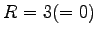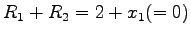## 4. 1. 2 Constants

One basic element of the algebraic structure a constant linear equation. It has to be noted that a constant symbol without any variable dependence does not have any sense, if interpreted as function, because, if the respective constant is non-zero, the equation always leads to a contradiction, e.g.. As a constant which is added to a linear or linearized residuum gives an expression which is sensible e.g., where it is possible to retrieve a proper solution. With respect to the matrix formulation, an additive constant expression influences the right hand side entry of the respective line or the equation system.

Michael 2008-01-16